# Engineering Mechanics - Friction

## Why Engineering Mechanics Friction?

In this section you can learn and practice Engineering Mechanics Questions based on "Friction" and improve your skills in order to face the interview, competitive examination and various entrance test (CAT, GATE, GRE, MAT, Bank Exam, Railway Exam etc.) with full confidence.

## Where can I get Engineering Mechanics Friction questions and answers with explanation?

IndiaBIX provides you lots of fully solved Engineering Mechanics (Friction) questions and answers with Explanation. Solved examples with detailed answer description, explanation are given and it would be easy to understand. All students, freshers can download Engineering Mechanics Friction quiz questions with answers as PDF files and eBooks.

## Where can I get Engineering Mechanics Friction Interview Questions and Answers (objective type, multiple choice)?

Here you can find objective type Engineering Mechanics Friction questions and answers for interview and entrance examination. Multiple choice and true or false type questions are also provided.

## How to solve Engineering Mechanics Friction problems?

You can easily solve all kind of Engineering Mechanics questions based on Friction by practicing the objective type exercises given below, also get shortcut methods to solve Engineering Mechanics Friction problems.

### Exercise :: Friction - General Questions

• Friction - General Questions
1.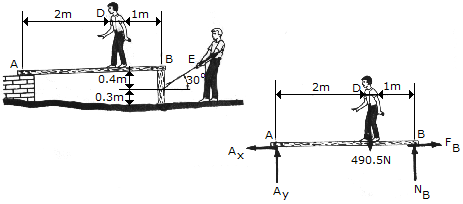The boy at D has a mass of 50 kg, a center of mass at G, and stands on a plank at the position shown. The plank is pin-supported at A and rests on a post at B. Neglecting the weight of the plank and post, determine the magnitude of force P his friend (?) at E must exert in order to pull out the post. Take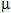B = 0.3 andC = 0.8.

 A. P = 360 N B. P = 264 N C. P = 229 N D. P = 293 N

Explanation:

No answer description available for this question. Let us discuss.

2.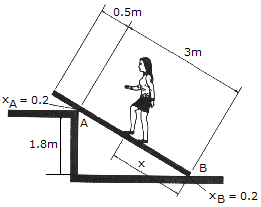A uniform beam has a mass of 18 kg and rests on two surfaces at points A and B. Determine the maximum distance x to which the girl can slowly walk up the beam before it begins to slip. The girl has a mass of 50 kg and walks up the beam with a constant velocity.

 A. x = 0.678 m B. x = 0.508 m C. x = 1.005 m D. x = 0.712 m

Explanation:

No answer description available for this question. Let us discuss.

3.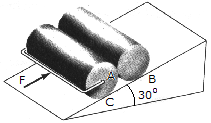Determine the minimum force F needed to push the two 75-kg cylinders up the incline. The force acts parallel to the plane and the coefficients of friction at the contacting surfaces areA = 0.3,B = 0.25,C = 0.4. Each cylinder has a radius of 150 mm.

 A. F = 919 N B. F = 735 N C. F = 1.051 kN D. F = 981 N

Explanation:

No answer description available for this question. Let us discuss.

4.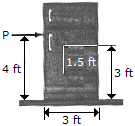The refrigerator has a weight of 200 lb and a center of gravity at G. Determine the force P required to move it. Will the refrigerator tip or slip? Take= 0.4.

 A. P = 75 lb Slips B. P = 80 lb Tips C. P = 80 lb Slips D. P = 75 lb Tips

Explanation:

No answer description available for this question. Let us discuss.

5.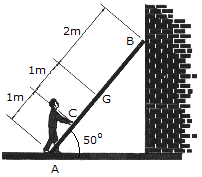A 17-kg ladder has a center of mass at G. If the coefficients of friction at A and B areA = 0.3 andB = 0.2, respectively, determine the smallest horizontal force that the man must exert of the ladder at point C in order to push the ladder forward.

 A. F = 120.2 N B. F = 288 N C. F = 166.8 N D. F = 204 N